Question

50.0 mL of a stock solution of hydrochloric acid, HCl (aq), at 12.0 M is diluted...

50.0 mL of a stock solution of hydrochloric acid, HCl (aq), at 12.0 M is diluted by adding it to 150.0 mL of water, H2O. What is the concentration, in units of molarity, of the final, diluted solution?

We need at least 10 more requests to produce the answer.

0 / 10 have requested this problem solution

The more requests, the faster the answer.

All students who have requested the answer will be notified once they are available.

Earn Coins

Coins can be redeemed for fabulous gifts.

Similar Homework Help Questions
• Q3.1 3 Points A 12.0 M stock solution of HCl(aq) is diluted with water to give...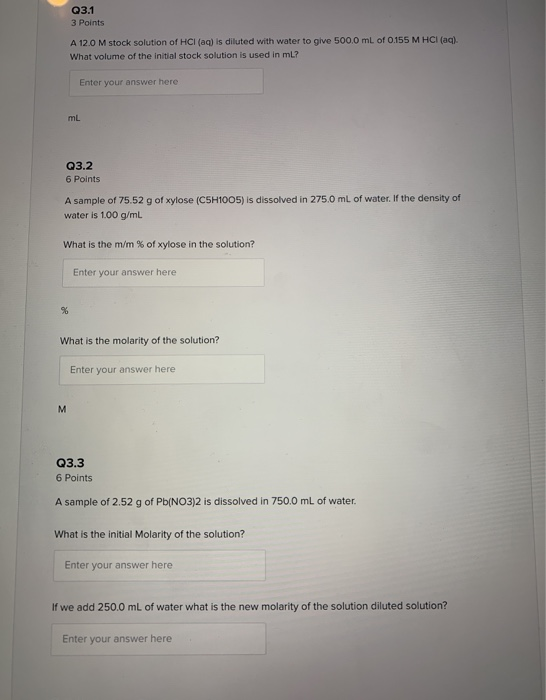Q3.1 3 Points A 12.0 M stock solution of HCl(aq) is diluted with water to give 500.0 ml of 0.155 M HCl(aq). What volume of the initial stock solution is used in ml? Enter your answer here mL Q3.2 6 Points A sample of 75.52 g of xylose (C5H1005) is dissolved in 275.0 mL of water. If the density of water is 1.00 g/ml What is the m/m % of xylose in the solution? Enter your answer here What is...

• If 32.12 mL of 3.77 M hydrochloric acid solution, HCl, are titrated with 51.5 mL of sodium hydroxide solution, NaOH...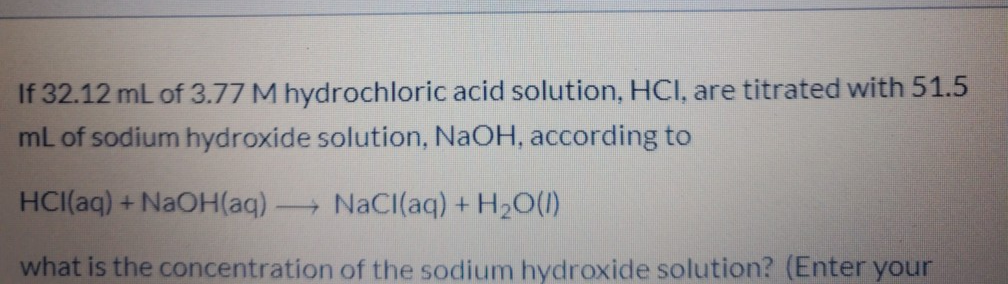If 32.12 mL of 3.77 M hydrochloric acid solution, HCl, are titrated with 51.5 mL of sodium hydroxide solution, NaOH, according to HCl(aq) + NaOH(aq) - NaCl(aq) + H2O(1) what is the concentration of the sodium hydroxide solution? (Enter your

• How many milliliters of 10.5 M HCl(aq) are needed to prepare 740.0 mL of 1.00 M...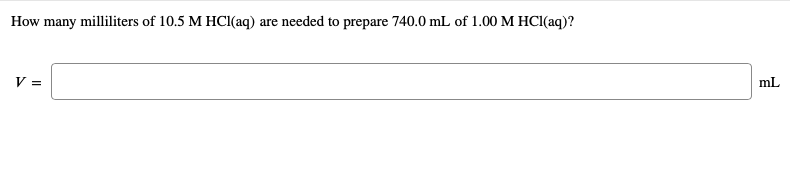How many milliliters of 10.5 M HCl(aq) are needed to prepare 740.0 mL of 1.00 M HCl(aq)? A 61.0 mL aliquot of a 1.20 M solution is diluted to a total volume of 238 mL. A 119 mL portion of that solution is diluted by adding 113 mL of water. What is the final concentration? Assume the volumes are additive. concentration:

• Question 355 pts 50.0 mL of a 3.00 M HCl solution is diluted to a total...

Question 355 pts 50.0 mL of a 3.00 M HCl solution is diluted to a total volume of 276.3 mL. What is the concentration of the diluted solution?

• Sodium carbonate, Na2COs, reacts with hydrochloric acid, HCl, to produce sodium chloride, carbon dioxide and water....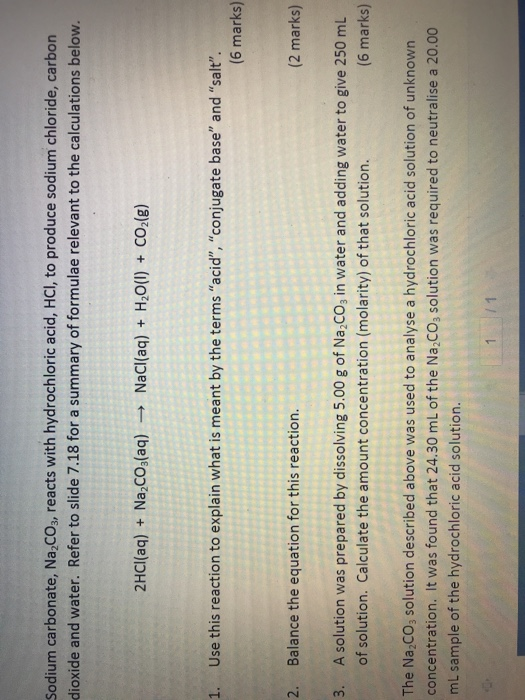Sodium carbonate, Na2COs, reacts with hydrochloric acid, HCl, to produce sodium chloride, carbon dioxide and water. Refer to slide 7.18 for a summary of formulae relevant to the calculations below. 2HCI(aq) Na,cO3(aq) NaCl(aq) H2O(I) CO2(g) 1. Use this reaction to explain what is meant by the terms "acid", "conjugate base" and "salt". (6 marks) 2 Balance the equation for this reaction. (2 marks) A solution was prepared by dissolving 5.00 g of Na,CO3 in water and adding water to give...

• Iron(III) hydroxide reacts hydrochloric acid via the following reaction: Fe(OH)3(aq)   +   2 HCl(aq)   ⇋   SrCl2(aq)   +  ...

Iron(III) hydroxide reacts hydrochloric acid via the following reaction: Fe(OH)3(aq)   +   2 HCl(aq)   ⇋   SrCl2(aq)   +   2 H2O(l) (5 pts) If the concentration of Fe(OH)­3 is 0.00684M, what is the pH of the iron(III)hydroxide solution? (8 pts) If 37.42 mL of 0.00684M iron(III)hydroxide is required to neutralize 25.00 mL of HCl, what is the concentration of the HCl solution in molarity?

• Sodium carbonate, Na2CO3, reacts with hydrochloric acid, HCl, to produce sodium chloride, carbon dioxide and water....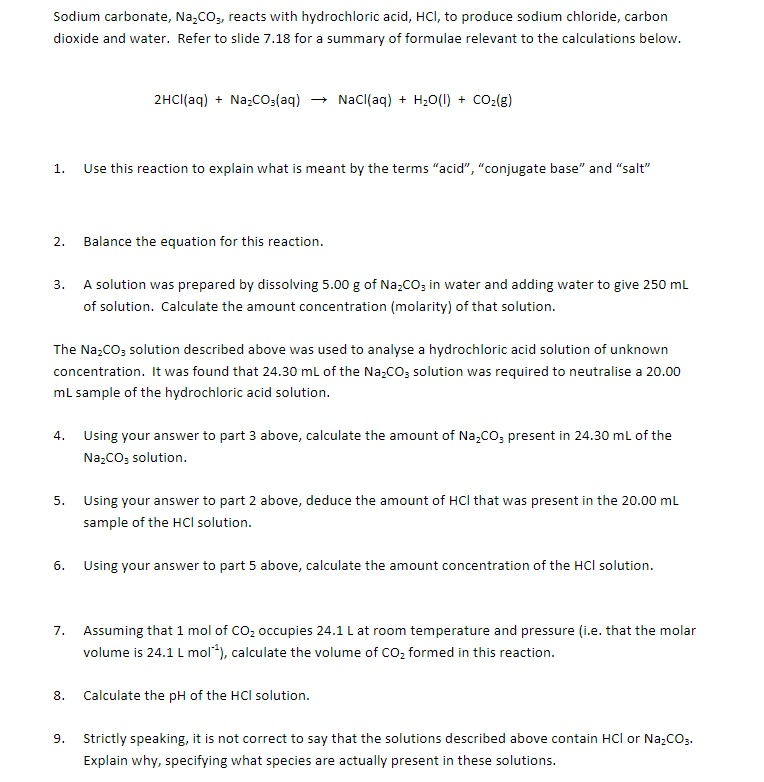Sodium carbonate, Na2CO3, reacts with hydrochloric acid, HCl, to produce sodium chloride, carbon dioxide and water. Refer to slide 7.18 for a summary of formulae relevant to the calculations below. 2HCl(aq) + Na.CO3(aq) + NaCl(aq) + H2O(l) + CO2(g) 1. Use this reaction to explain what is meant by the terms "acid", "conjugate base" and "salt" 2. Balance the equation for this reaction. 3. A solution was prepared by dissolving 5.00 g of Na2CO3 in water and adding water to...

• You wish to make a 0.249 M hydrobromic acid solution from a stock solution of 12.0...

You wish to make a 0.249 M hydrobromic acid solution from a stock solution of 12.0 M hydrobromic acid. How much concentrated acid must you add to obtain a total volume of 50.0 mL of the dilute solution? __mL You wish to make a 0.112 M nitric acid solution from a stock solution of 12.0 M nitric acid. How much concentrated acid must you add to obtain a total volume of 150 mL of the dilute solution? __mL In the...

• In the laboratory, you dilute 3.61 mL of a concentrated 12.0 M hydrochloric acid solution to...

In the laboratory, you dilute 3.61 mL of a concentrated 12.0 M hydrochloric acid solution to a total volume of 175 mL. What is the concentration of the dilute solution?

• 6) A stock solution of HCl is 11.50 M, if 15.29 ml of the stock solution...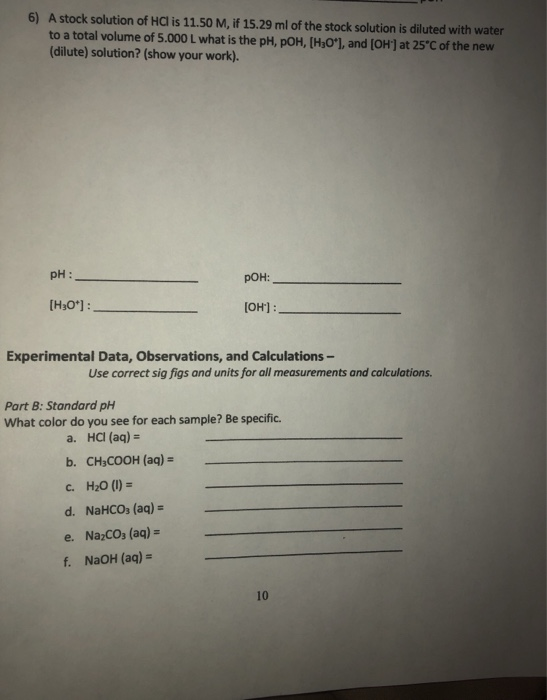6) A stock solution of HCl is 11.50 M, if 15.29 ml of the stock solution is diluted with water to a total volume of 5.000 L what is the pH, POH, [H3O*], and (OH) at 25°C of the new (dilute) solution? (show your work). pH : рон: [H30*) : [OH') : Experimental Data, Observations, and Calculations - Use correct sig figs and units for all measurements and calculations Part B: Standard pH What color do you see for each...